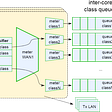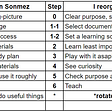# Opening

Easy

Given an array `arr`, replace every element in that array with the greatest element among the elements to its right, and replace the last element with `-1`.

After doing so, return the array.

Example 1:

`Input: arr = [17,18,5,4,6,1]Output: [18,6,6,6,1,-1]`

Constraints:

• `1 <= arr.length <= 10^4`
• `1 <= arr[i] <= 10^5`

# Solution

This is a “tough” easy problem because the example isn’t exactly clear and there is only one example. Here are the facts.

We have to replace every element in the array with the greatest element to the right. Then we have to replace the last element with -1. After doing so, we return the solution.

First thing first, you should be able to loop through an entire array. While doing so, you should keep track of the numbers that you are on.

Using the example, [17,18,5,4,6,1], we should return [18,6,6,6,1,-1]. We loop through this array and see 17, to the right which is the next number is 18 which is greater.

18 replaces 17. Go to the next number, we have 5. 5 is bigger than 4, move onto the next number. 5 is smaller than 6, 6 replaces the last two numbers. 1 is the last number, then we put -1.

This is convoluted. In Python, you could effectively do this in three lines. It could look like this:

`class Solution:    def replaceElements(self, arr: List[int]) -> List[int]:        mx = -1        for i in range(len(arr) - 1, -1, -1):            arr[i], mx = mx, max(arr[i], mx)        return arr`

This solution starts at the end of the list. You start with setting a variable called mx or max to -1. Using the range() function, you are saying to start at the end and iterate through each one.

The range() function has three parameters: start, stop, step

You start at index 5 (if we are using the example), stop right at 0, going down one step at a time. As you do so, you set arr[i] to the mx, which at the first iteration is -1. Then you set mx to the max of (arr[i], mx).

That is, the max of (1, -1). 1 is bigger, so you set mx = 1 and move to the next iteration. Doing this will yield a O(n) because you have to go through each element no matter what.

The easier to code method is O(n²) because you will run two loops. The first loop is where you iterate one at a time, the second loop to iterate through each number to its right until you find the biggest number.

Once you have the biggest number, all the number you just landed on will be replaced by the biggest number. Because the last number doesn’t have a number to its right, you replace it with -1.

Hope this helps.

--

--

--

I write just in case death knocks

Love podcasts or audiobooks? Learn on the go with our new app.

## Advanced QoS in vRouter Fast Path## Filecoin Payment Bridge## Soft skills for a normal programmer — Part 1. Meta-learning: How to learn to complete your project## Setup Oracle Database 12c image on Docker — Step by Step## Basics of GUI in Python## Cassy

I write just in case death knocks

## Leetcode: Dynamic sliding window pattern